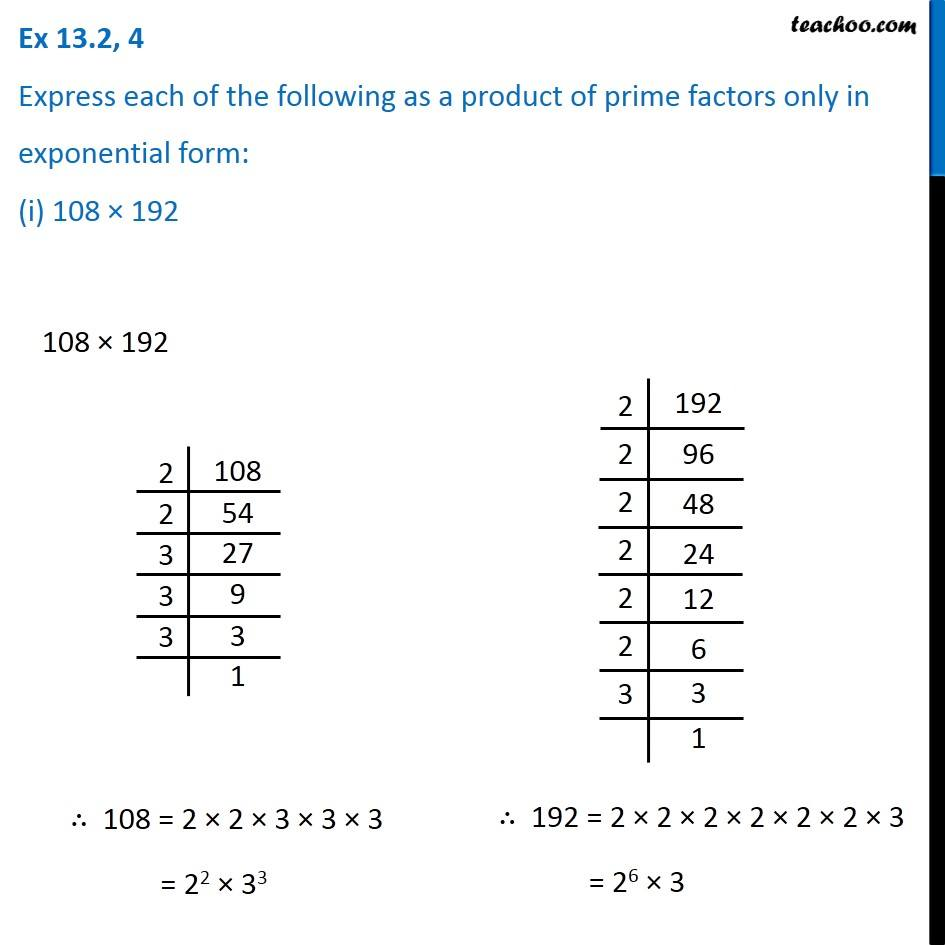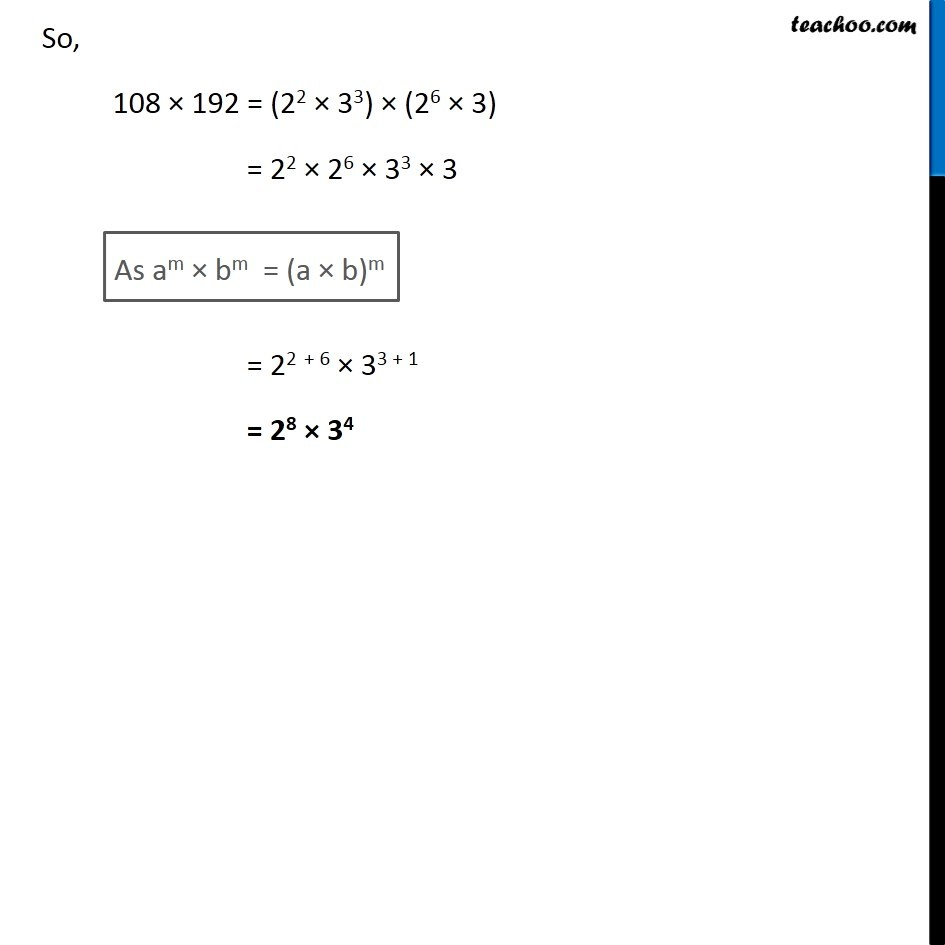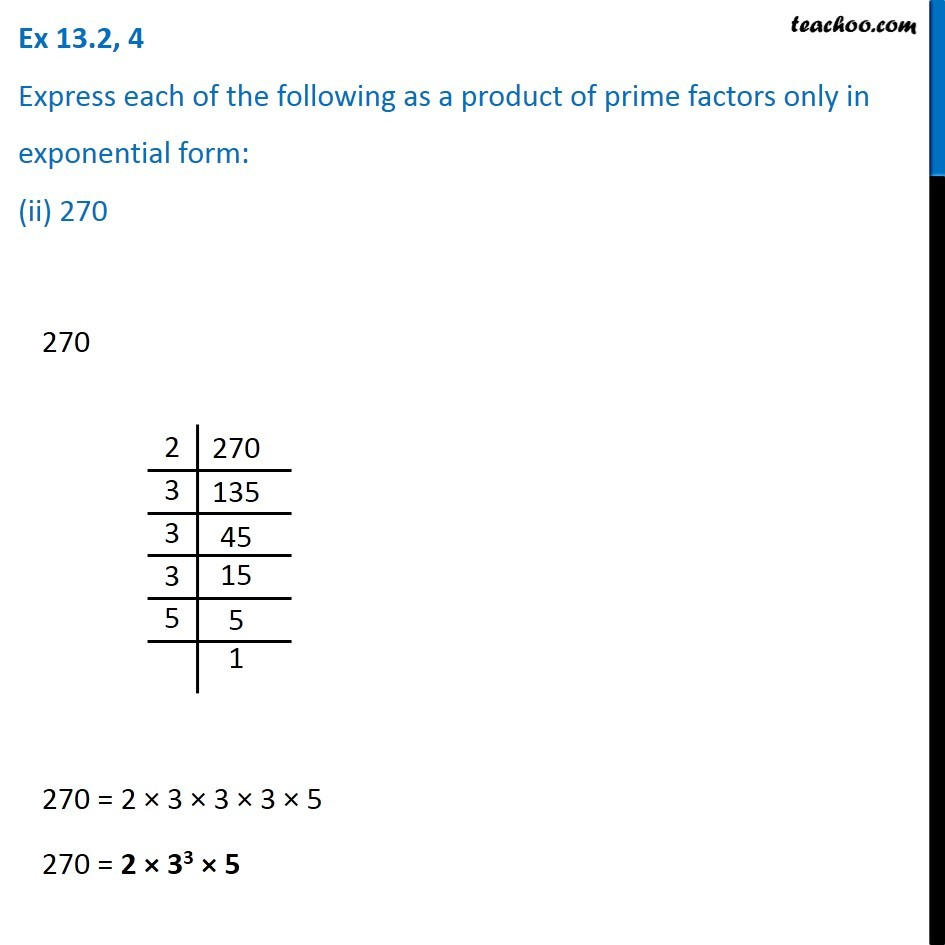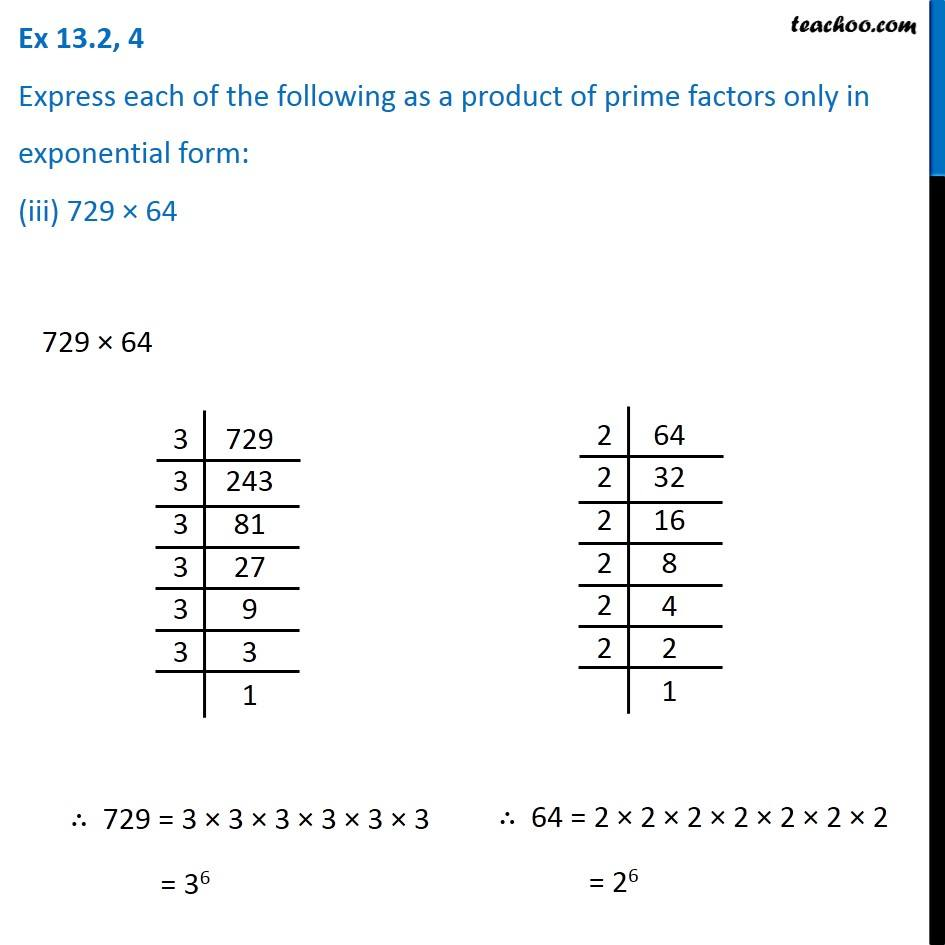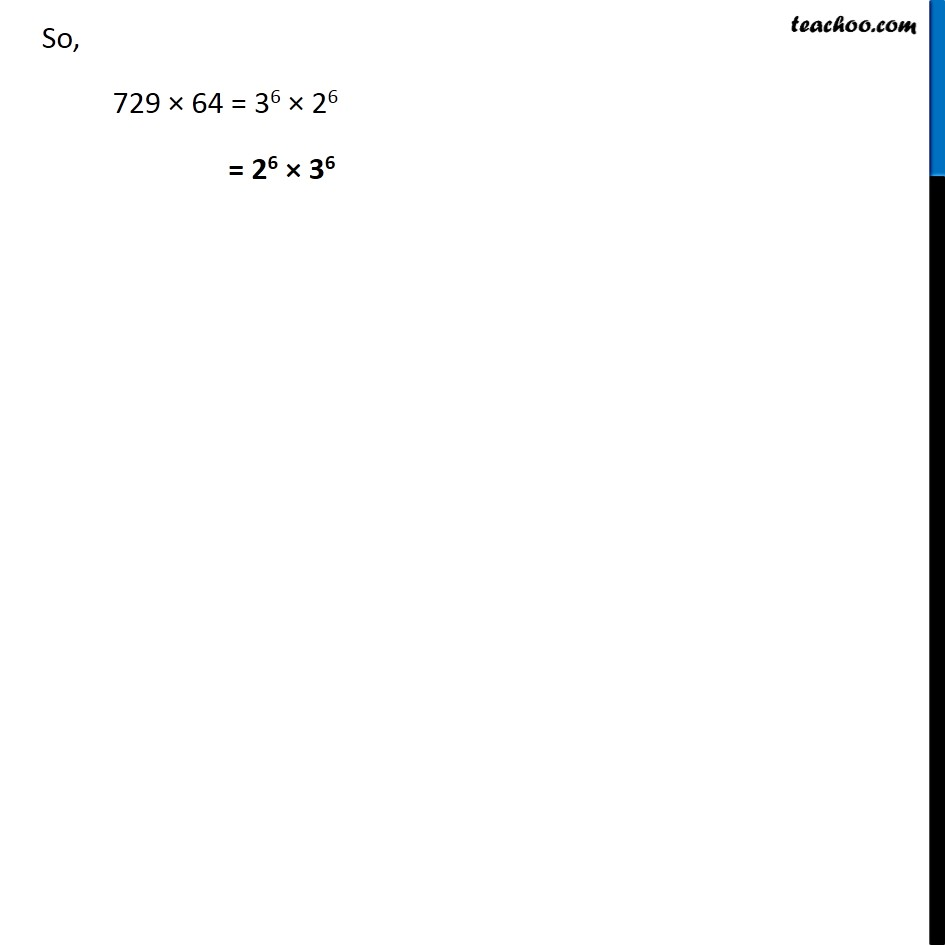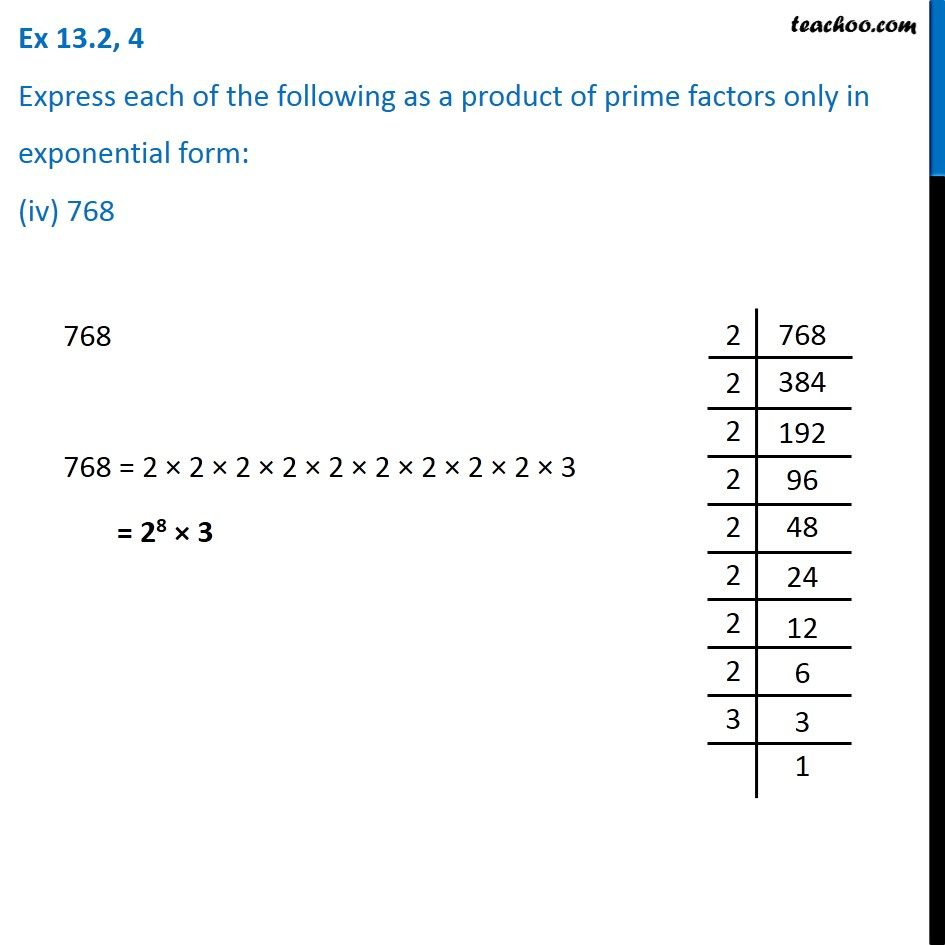1. Chapter 13 Class 7 Exponents and Powers
2. Serial order wise
3. Ex 13.2

Transcript

Ex 13.2, 4 Express each of the following as a product of prime factors only in exponential form: (i) 108 × 192 108 × 192 2|108 2|54 3|27 3|9 3|3 |1 2|192 2|96 2|48 2|24 2|12 2|6 2|3 |1 ∴ 108 = 2 × 2 × 3 × 3 × 3 = 22 × 33 ∴ 192 = 2 × 2 × 2 × 2 × 2 × 2 × 3 = 26 × 3 So, 108 × 192 = (22 × 33) × (26 × 3) = 22 × 26 × 33 × 3 As am × bm = (a × b)m = 22 + 6 × 33 + 1 = 28 × 34 Ex 13.2, 4 Express each of the following as a product of prime factors only in exponential form: (ii) 270 270 2|270 3|135 3|45 3|15 3|5 |1 270 = 2 × 3 × 3 × 3 × 5 270 = 2 × 33 × 5 Ex 13.2, 4 Express each of the following as a product of prime factors only in exponential form: (iii) 729 × 64 729 × 64 3|729 3|243 3|81 3|27 3|9 3|3 |1 2|64 2|32 2|16 2|8 2|4 2|2 |1 ∴ 729 = 3 × 3 × 3 × 3 × 3 × 3 = 36 ∴ 64 = 2 × 2 × 2 × 2 × 2 × 2 × 2 = 26 So, 729 × 64 = 36 × 26 = 26 × 36 Ex 13.2, 4 Express each of the following as a product of prime factors only in exponential form: (iv) 768 768 768 = 2 × 2 × 2 × 2 × 2 × 2 × 2 × 2 × 2 × 3 = 28 × 3 2|768 2|384 2|192 2|96 2|48 2|24 2|12 2|6 3|3 |1

Ex 13.2